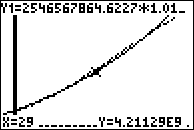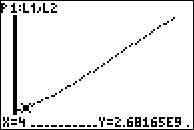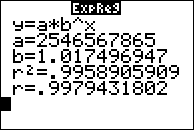# Activities

••• ##### Subject Area

• Math: Precalculus: Exponential and Logarithmic Functions

• ##### Author9-12

30 Minutes

• ##### Device
• TI-83 Plus Family
• TI-84 Plus
• TI-84 Plus Silver Edition

## World Population#### Activity Overview

In this activity, students use their graphing calculator to explore world population data from the years 1950-2006. They will develop various equations to model the data.

#### Key Steps

•Students will use the calculator program WORLDPOP to get world population data on to their graphing calculator.

•Students will create a scatter plot to model the data.

•Students will find an exponential regression equation using the ExpReg command to fit the data.